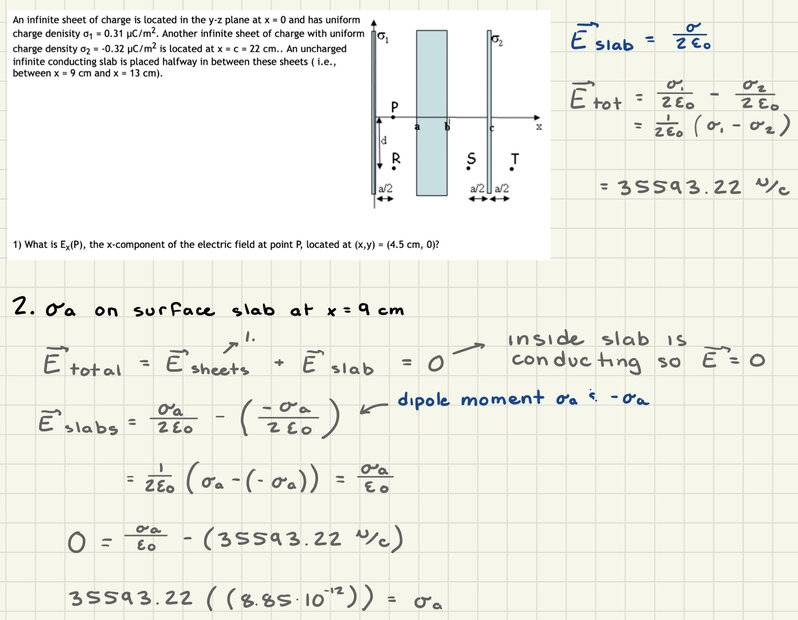# Finding Charge Density on the Surface of a Slab

• LulaBell

#### LulaBell

Homework Statement
An infinite sheet of charge is located in the y-z plane at x = 0 and has uniform charge density σ1 = 0.31 μC/m2. Another infinite sheet of charge with uniform charge density σ2 = -0.32 μC/m2 is located at x = c = 22 cm. An uncharged infinite conducting slab is placed halfway in between these sheets ( i.e., between x = 9 cm and x = 13 cm).

What is σa, the charge density on the surface of the conducting slab at x = 9 cm?
Relevant Equations
E infinite slab = σ/2Eo
Here's my attempt at a solution, but when I plug it in, it gives me a power ten error. I don't really understand what I'm doing wrong here. I think all my variables are in the correct units and it asks for my answer to be in μC/m2. Any help is much appreciated.Homework Statement:: An infinite sheet of charge is located in the y-z plane at x = 0 and has uniform charge density σ1 = 0.31 μC/m2. Another infinite sheet of charge with uniform charge density σ2 = -0.32 μC/m2 is located at x = c = 22 cm. An uncharged infinite conducting slab is placed halfway in between these sheets ( i.e., between x = 9 cm and x = 13 cm).

What is σa, the charge density on the surface of the conducting slab at x = 9 cm?
Relevant Equations:: E infinite slab = σ/2Eo

Here's my attempt at a solution, but when I plug it in, it gives me a power ten error. I don't really understand what I'm doing wrong here. I think all my variables are in the correct units and it asks for my answer to be in μC/m2. Any help is much appreciated.

View attachment 322397
You don't say what your final answer is. It should be obvious it will be similar in magnitude to the two given charge densities.

•MatinSAR
My final answer was 3.15 * 10^-7 which was wrong so I thought maybe it was supposed to be 3.15 uC/m^2 but that was wrong as well.

My final answer was 3.15 * 10^-7 which was wrong so I thought maybe it was supposed to be 3.15 uC/m^2 but that was wrong as well.
Neither of those is the same order of magnitude as the two given charge distributions, so must be wrong.